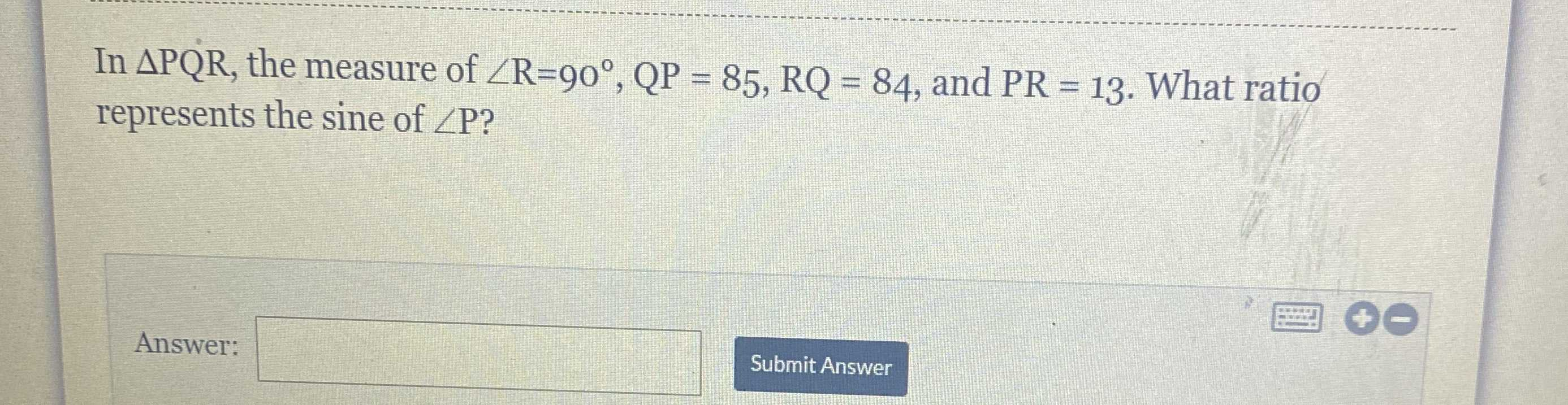### ¿Todavía tienes preguntas de matemáticas?

Pregunte a nuestros tutores expertos
Trigonometry
PreguntaIn $$\triangle PQR$$ , the measure of $$\angle R = 90 ^ { \circ } , QP = 85 , RQ = 84$$ , and $$PR = 13$$ . What ratio represents the sine of $$\angle P$$ ? Answer:

81$$°$$